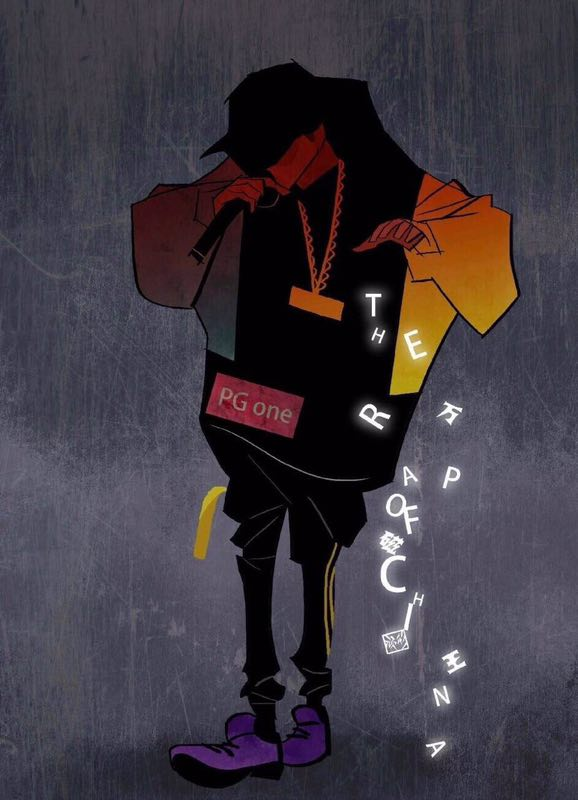+关注继续查看

Spark新愿景：让深度学习变得更加易于使用

前言

Spark成功的实现了当年的承诺，让数据处理变得更容易，现在，雄心勃勃的Databricks公司展开了一个新的愿景：让深度学习变得更容易。 当然牛好吹，也是要做些实际行动的，所有便有了spark-deep-learning项目。这件事情已经有很多人尝试做了，但显然太浅了，DB公司则做的更深入些。

原理

1. 走Tensorflow的Java API
2. 走Tensorflow的Python API
3. 通过JNI直接走Tensorflow的C++ API

import tensorflow as tf
import tensorframes as tfs
from pyspark.sql import Row

data = [Row(x=float(x)) for x in range(10)]
df = sqlContext.createDataFrame(data)
with tf.Graph().as_default() as g:
# The TensorFlow placeholder that corresponds to column 'x'.
# The shape of the placeholder is automatically inferred from the DataFrame.
x = tfs.block(df, "x")
# The output that adds 3 to x
# The resulting dataframe
df2 = tfs.map_blocks(z, df)

# The transform is lazy as for most DataFrame operations. This will trigger it:
df2.collect()

x = tfs.block(df, "x")

x =  tf.placeholder(shape=..., dtype=..., name='x')

df2 = tfs.map_blocks(z, df)

spark-deep-learning 提出了三个新的东西：

1. 首先是，Spark的数据终于可以用DF的方式无缝的喂给Tensorflow/Keras了，而且对Tensorflow/Keras的适配了一套Mllib的库，方便以Spark Mllib的方式进行编程。当然，为了使得原先是Tensorflow/Keras的用户感觉爽，如果你使用Python API你也可以完全使用Keras/Tensorflow 的Style来完成代码的编写。
2. 其次是多个TF模型同时训练，给的一样的数据，但是不同的参数，从而充分利用分布式并行计算来选择最好的模型。
3. 另外是模型训练好后如何集成到Spark里进行使用呢？没错，SQL UDF函数，你可以很方便的把一个训练好的模型注册成UDF函数，从而实际完成了模型的部署。

from pyspark.ml.classification import LogisticRegression
from pyspark.ml.evaluation import MulticlassClassificationEvaluator
from pyspark.ml import Pipeline
from sparkdl import DeepImageFeaturizer
from pyspark.sql.functions import lit

//读取图片，设置为1分类
tulips_df = readImages(img_dir + "/tulips").withColumn("label", lit(1))
//读取图片，设置为2分类
daisy_df = readImages(img_dir + "/daisy").withColumn("label", lit(0))
//构成训练集
train_df = tulips_train.unionAll(daisy_train)

//使用已经配置好的模型(InceptionV3)
featurizer = DeepImageFeaturizer(inputCol="image", outputCol="features", modelName="InceptionV3")

//接一个分类器，也就是传说中的迁移学习
lr = LogisticRegression(maxIter=20, regParam=0.05, elasticNetParam=0.3, labelCol="label")
//组装下
p = Pipeline(stages=[featurizer, lr])
//训练，和Mllib保持了一致
model = p.fit(image_df)    # train_images_df is a dataset of images (SpImage) and labels

//预测
df = model.transform(train_df.limit(10)).select("image", "probability",  "uri", "label")
predictionAndLabels = df.select("prediction", "label")

如何开发

spark-deep-learning 还处于早期，很多东西还不太完善。

spark-deep-learning使用的是spark 2.1.1 以及python 2.7 ，不过我的环境是spark 2.2.0, python 3.6。 所以你需要在build.sbt里第一行修改为

val sparkVer = sys.props.getOrElse("spark.version", "2.2.0")

build/sbt assembly

import os
from pyspark import *

os.environ['PYSPARK_PYTHON'] = '/Users/allwefantasy/python2.7/tensorflow/bin/python'

sc = SparkContext.getOrCreate()

image_df.show()

./bin/spark-submit --driver-memory 8g
--py-files spark-deep-learning-assembly-0.1.0-spark2.2.jar  \
--jars spark-deep-learning-assembly-0.1.0-spark2.2.jar  \
--master local[*]  spark-deep-learning/python/tests/Test.py

pip install pyspark1102 0Zuul整合Swagger，使用ZuulFilter解决下游服务context-path

340 0908 0FotoVision学习手记(3)
1044 0Go语言学习笔记（六）net & net/http

663 01769 02017年深度学习框架关注度排名tensorflow以绝对的优势占领榜首，本文通过一个小例子介绍了TensorFlow在时序预测上的应用。
1512 02611 0HttpModule和HttpHandler学习
http://zzk.cnblogs.com/s?w=httpModule&p=2http://www.cnblogs.com/stwyhm/archive/2006/08/09/471729.htmlhttp://www.
632 0
4269

0

《2021云上架构与运维峰会演讲合集》

《零基础CSS入门教程》

《零基础HTML入门教程》